# CBSE Previous Year Questions: Polynomials Notes | Study Mathematics (Maths) Class 10 - Class 10

## Class 10: CBSE Previous Year Questions: Polynomials Notes | Study Mathematics (Maths) Class 10 - Class 10

The document CBSE Previous Year Questions: Polynomials Notes | Study Mathematics (Maths) Class 10 - Class 10 is a part of the Class 10 Course Mathematics (Maths) Class 10.
All you need of Class 10 at this link: Class 10

Q.1. Find the quadratic polynomial, sum and product of whose zeroes are -1 and -20 respectively. Also, find the zeroes of the polynomial so obtained.

[CBSE 2019, (30/4/2)]
Ans: Let α and β be the zeroes of the quadratic polynomial.
∴ Sum of zeroes, α + β = - 1
and Product of zeroes, α . β = - 20
= x2-(α + β). x + αβ
= x2 - ( - 1 ) x - 20
= x2 + x - 20
Now, for zeroes of this polynominal: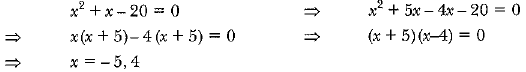⇒ x = - 5,4
∴ zeroes are - 5 and 4

Q.2. Find the zeroes of the quadratic polynomial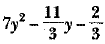and verify the relationship between zeroes and the coefficients.    [CBSE 2019, (30/2/1)]
Ans: Given: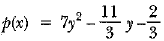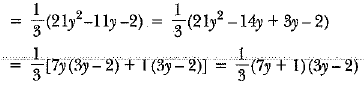Equating p(x) = 0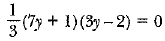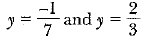Now, the sum of zeroes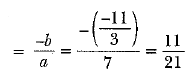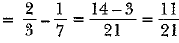and product of zeroes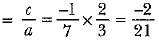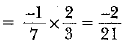Hence verified

Q.3. If α and β are the zeroes of the quadratic polynomial f(x)  = x2- 4x +3. find the value of α4 β2 + α2 β4    [CBSE 2019, (30/4/1)]
f(x) = x2 - 4x + 3
∴ Sum of zeroes =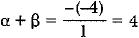⇒ α + β = 4
and product of zeroes,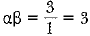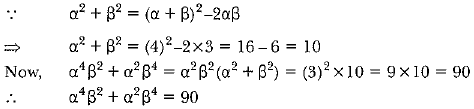Q.4. Find the value of k such that the polynomial. x2 - (k + 6)x + 2(2k - 1) has sum of its zeroes equal to half of their product.    [Delhi 2019]
Ans: The given polynomial is
= x2 - (k + 6)x + 2(2k - 1)
Let α and β be the zeroes of polynomial.
So,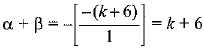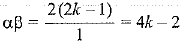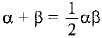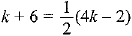⇒  2k + 12 = 4k - 2
⇒ 2k = 14 ⇒ k = 7

Q.5. Find all zeroes of the polynomial 3x3+ 10x2- 9x- 4 if one of its zero is 1.

[Delhi 2019]
Ans: 1 is one of the zero of p(x) = 3x3 + 10x2 - 9x - 4
⇒ x = 1 satisfies the polynomial p(x)
∴ (x - 1) is a factor of p(x)
Hence (x - 1) divides p(x) completely.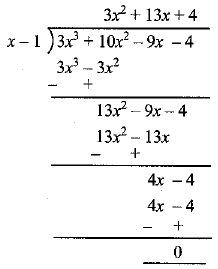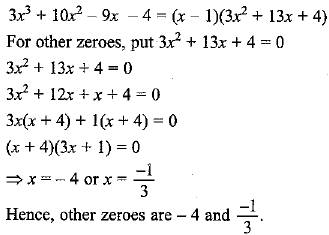Q.6. Find all zeroes of the polynomial (2x4 - 9x3 + 5x2 + 3x - 1) if two of its zeroes are (2 + √3) and (2 - √3).    [CBSE 2018]
Ans: Since, (2 + √3) and (2 - √3) are the two zeroes of the given polynomial  2x4 - 9x3 + 5x2 + 3x - 1,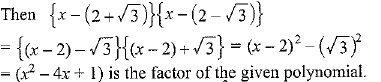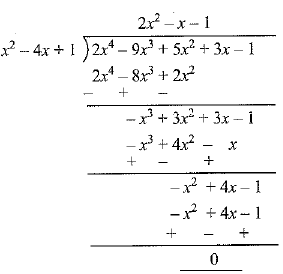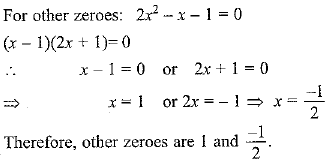Q.8. If α find β are zeroes of the quadratic polynomial 4x2 + 4x + 1, then form a quadratic polynomial whose zeroes are 2α and 2β.    [CBSE 2015]
Ans: p(x) = 4x2 + 4x + 1
∵ α,β are  zeroes of p(x)
∴  α + β = sum of zeroes = -b/a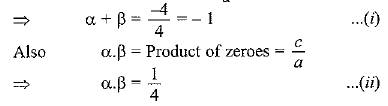Now a quadratic polynomial whose zeroes are 2α and 2β.
x2 - (sum of zeroes)x + Product of zeroes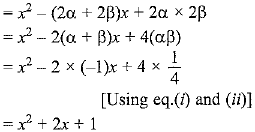Q.1. If one zero of the quadratic polynomial f(x) = 4x2 - 8kx + 8x - 9 is negative of the other, then find zeroes of kx2 + 3kx + 2.    [CBSE 2015]
Ans: f(x) = 4x2 - 8kx + 8x - 9 = 4x2 + (8 - 8k)x - 9
Let one zero = α
∴ other zero = -α
Now Sum of zeroes =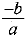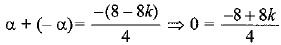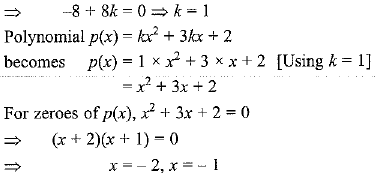The document CBSE Previous Year Questions: Polynomials Notes | Study Mathematics (Maths) Class 10 - Class 10 is a part of the Class 10 Course Mathematics (Maths) Class 10.
All you need of Class 10 at this link: Class 10Use Code STAYHOME200 and get INR 200 additional OFF

## Mathematics (Maths) Class 10

58 videos|354 docs|138 tests

Track your progress, build streaks, highlight & save important lessons and more!

,

,

,

,

,

,

,

,

,

,

,

,

,

,

,

,

,

,

,

,

,

;